# Difference between revisions of "Carmichael number"

A composite natural numberfor which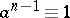modulo, wheneveris relatively prime to. Thus they are pseudo-primes (cf. Pseudo-prime) for every such base. These numbers play a role in the theory of probabilistic primality tests (cf. Probabilistic primality test), as they show that Fermat's theorem, to wit $a^p \equiv a$ modulo, wheneveris prime andmodulo, is not a sufficient criterion for primality (cf. also Fermat little theorem).

The first five Carmichael numbers are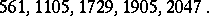R.D. Carmichael [a2] characterized them as follows. Letbe the exponent of the multiplicative group of integers modulo, that is, the least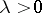making allth powers in the group equal to. (This is readily computed from the prime factorization of.) Then a composite natural numberis Carmichael if and only if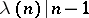. From this it follows that every Carmichael number is odd, square-free, and has at leastdistinct prime factors.

Let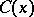denote the number of Carmichael numbers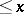. W.R. Alford, A. Granville and C. Pomerance [a1] proved that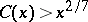for sufficiently large. This settled a long-standing conjecture that there are infinitely many Carmichael numbers. It is believed on probabilistic grounds that[a4].

There is apparently no better way to computethan to make a list of the Carmichael numbers up to. The most exhaustive computation to date (1996) is that of R.G.E. Pinch, who used the methods of [a3] to determine that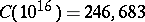.

How to Cite This Entry:
Carmichael number. Encyclopedia of Mathematics. URL: http://encyclopediaofmath.org/index.php?title=Carmichael_number&oldid=15179
This article was adapted from an original article by E. Bach (originator), which appeared in Encyclopedia of Mathematics - ISBN 1402006098. See original article